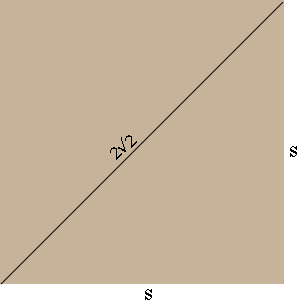SEARCH HOMEMath Central Quandaries & QueriesQuestion from kalyani, a student: the curved surface of a cylinder is a square of diagonal 2*square root of 2. What is the area of the base of the cylinder?Hi Kalyani,

Suppose the length of the side of the square is $S$ units.What is $S?$ Maybe Pythagoras can help.

When the square is rolled up to form a circular cylinder the bade is a disk with circumference $S$ units. What is its radius? What is its area?

PennyMath Central is supported by the University of Regina and the Imperial Oil Foundation.